# Fourier transform of Bessel function

bobred

## Homework Statement

Noting that $J_0(k)$ is an even function of $k$, use the result of part (a) to
obtain the Fourier transform of the Bessel function $J_0(x)$.

## Homework Equations

In (a) I am asked to show that the Fourier transform of
$$f(x)=\dfrac{1}{\sqrt{1-x^{2}}}$$
is
$$\tilde{f}(k)=\sqrt{\pi/2}J_0(-k)$$
where
$$J_0(x)=\frac{1}{\pi}\int_{0}^{\pi} e^{i x \cos \theta}d \theta$$

## The Attempt at a Solution

I have found the Fourier transform of $f(x)$ using trig substitution I just cant see how to get the FT of $J_0(x)$.
Any hints as to where I should begin?

## Answers and Replies

Fightfish
Have you heard of the Fourier inversion theorem?
Make use of that, and the hint that question provided about the even nature of the Bessel function.

•bobred
bobred
Hi
I went over my notes a few times and got it.
Thanks

bobred
Considering the second derivative of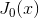show the Fourier transform of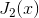is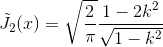I have done similar for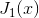using rules for derivatives of Fourier transforms but can't see where to start, where the numerator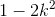comes from.